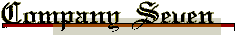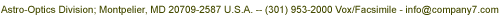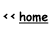C-7 Home PageC-7 NewsConsignmentLibraryProducts & ServicesProduct LinesOrderSearch C7.com

#### Addendum to the Set-Up of the Vixen "Sky Sensor III" Computer

This sheet has evolved to better explain the instruction manual, especially Section 1 "Initial Setting" of the "Sky Sensor III" computer as several customers have asked for a clarification of step number 8, on page 16 of the instruction manual.

Please note that to input any number or command into the "Sky Sensor III" the number or command must be followed by pressing the "CR" key.

Local Longitude:

The longitude of your observation site, this is easily determined by looking at an atlas and looking for the longitude (the North-South line) which is usually given in 1 degree increments. You may have an atlas that further breaks down the division by minutes, these will usually show as five to fifteen minute divisions. Remember there are 60 minutes in one degree of longitude or latitude.

Standard Longitude:

Standard Longitude is also known as "Standard Meridian". These are the longitude lines which are exactly divisible by 15 degrees. Examples of the Standard Longitudes are : 15, 30, 45, 60, 75, 90, 105, 120 and so on.

The nearest 15 degree increment of longitude is what must be known before setting up the Sky Sensor computer.

For example, Washington D.C. is at about 77 degrees West (west of Greenwich, England which is the zero line of longitude). This 77 degrees coordinate is 2 degrees West of the 75 degree line of longitude (the nearest 15 degree increment of longitude). Therefore, if we subtract 75 from 77 we get a difference of +2 degrees, so you would input "02" for the minutes difference when the "Sky Sensor III" asks for "LOG DEF DEG".

If we were at 72 degrees longitude, we would subtract 72 from 75 and get -3 so you would input "-03" for the minutes. It is important to input the R - R indicator if this is a negative number, otherwise the Sky Sensor will assume a positive number.

This is not essential but for better accuracy you should estimate or otherwise ascertain this difference in whole degrees, and in fractions of degrees. Degrees are divided by minute increments. There are 60 minutes in one degree.

For example: If you are at Longitude 76 degrees and 45 minutes (usually printed as 76 degrees 45 minutes), you would subtract 76 degrees 45 minutes from 75 degrees 00 minutes. The result is 1 degree 45 minutes. This would then be entered into the "Sky Sensor III". If you are at Longitude 72 degrees 10 minute, we subtract 72 degrees 10 minutes from 75 and get -3 degrees 50 minutes. Therefore you would input "-3".

This information may also be of assistance to those using the prior "Sky Sensor" models.

Thank you, and we wish you a lifetime of enjoyment with your telescope.